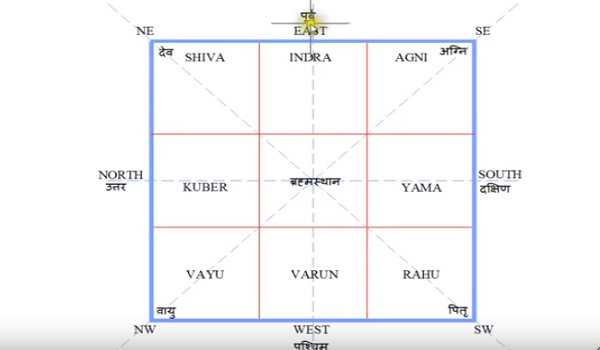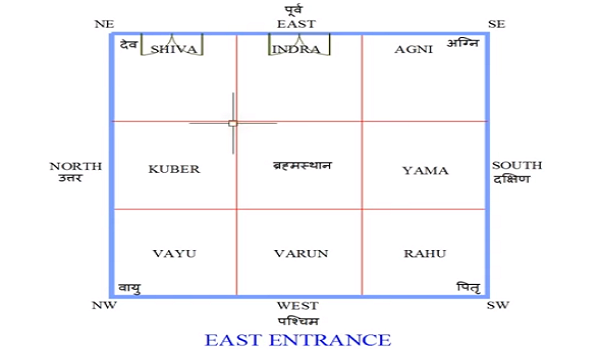# Basic Vastu Tips for 30 feet by 30 East Facing Plot

0
944

We are discussing on Vastu. How can we correct the architecture structure of the new plot?  Now we are discussing everything about Vaastu which will be typical. If you want to know about the correct architecture of the house according to Vaastu. Here we can see the diagram of the architectural structure of the plot. The size of the plot is represented in a square, Suppose it is 30 by 30 size of the plot. It is the most important thing to understand in the Vaastu so that we divide this plot into three by three parts through the red line. This plot contains nine section divide into three parts. It is easy to understand in which the position of the side is for whom and what will be the most first. We talk about the direction of the plot.Our four direction is east, west, north, south. Now we discuss sub direction are northeast, Southeast, northwest, and southwest all these sub-directions are separated. Now we discuss these directions. We will tell you two trick to find out the direction of the plot. Friends, firstly we find out the way to determine the direction of the plot. In order to find out the direction of the plot, we will tell you two options. Firstly we see the east direction of the plot. Anyone can tell the sun comes out from the east. If someone asks where the sun sets in, then you say west. For this purpose, you go to the plot and turn your face toward the east and get the sun from where the sun rises. Then your left-hand shows the direction of north and your right-hand shows the direction of the south. You have another option to find out the direction of the plot by using the compass. The compass always marks the left direction. Again, you are standing at the center point. Your left-hand side represents the north direction. Your face represents the east direction then your backside represents the west direction. After that, your right-hand side represents the south direction.Now, we talk about the ninth section of the map. The first section is of Shiva section, The second section is of Indra, The third section is of Agni dev says that the center of north is of Kuber, center of south section is of age, the seventh section is of Shiva, the eighth section is of Varuna, ninth section is of Rahu, and the central location of a map indicates the Brahmasthan. It is difficult to find a space in Delhi like the city, but it is necessary to leave the little bit space in the middle of the plot. We also represent the slope of the plot. I have been marked here.

Southwest corner of the map is a little bit high on the map. We can also make a variation. Minimum you take two-inch variation. Suppose you take the variation of 6 inches in southwest then you take two inches than two inches. Completely you get four inches of variation in the left side of the southwest. Whenever you cast a floor it should be done in the same manner as represented in the map.